ANALYSIS

Introduction
In analysing results, there are some commonly used statistical methods which enable us to summarise our results. Some of these methods require a great deal of complex analysis and thus consultation with an experienced statistician is recommended, however others can be done without consulting a statistician.

The analysis that is to be carried out should be taken into account at the planning stage of the survey. Even at that early stage, the output tables should be specified and the analysis techniques decided upon to ensure that the necessary data is collected.

Once the required data from our survey has been gathered, we are in a position to carry out the analysis of our results. This involves interpreting the data, breaking it down and manipulating it to answer the survey objectives. From here the data is sorted into categories and summarised to obtain information like descriptive statistics, frequencies, percentages, correlations and measures of both location and spread. This information is used to formulate hypotheses and make inferences about our population data by estimating confidence intervals and testing the hypotheses to determine significances and trends in our data.

A useful method in analysing data involves 'getting to know it' through the use of graphical methods and summary statistics. Exploratory data analysis enforces the importance of understanding the data before determining any suitable analyses. Summarising the data using graphics and summary statistics gives a clear, immediate picture of it and reveals any key relationships between variables.

DESCRIPTIVE STATISTICS
Descriptive statistics are summary measures which are calculated from observations in the population and are used to provide information about the distribution of particular variables in the population. In the case of responses from a sample of the population, the statistics are used to make inferences about the population.

Condensing Data

Forming Classes
One of the first things that needs to be done in analysing a set of observations from a population or sample is to condense the information into a more meaningful and manageable form. One fairly simple way of getting a feel for the data is to calculate a frequency distribution of responses and produce a histogram. When the data is quantitative (eg. age, income) it is necessary to group observations together into appropriate classes. The boundaries of the class intervals must be non-overlapping so that each observation can be allocated to only one interval. Obviously, the larger the class, the more information that is lost. The number of classes and the appropriate width of class interval are best determined by looking at the range of values in the data. A point worth noting is that the resulting frequency distribution is more readily comprehended if the class intervals are of equal width.

Frequency Tables and Cross-Tabulations
A common starting point in summarising data is producing frequency counts. These simply show the number of responses for each class of the variable of interest. Frequency tables can also be used to summarise the data enabling the reader to draw his/her own conclusions. Tables can be produced for both quantitative (eg. age, income) and categorical variables (eg. sex, occupation). The data below gives an illustration of the way in which frequency counts may be used to simplify a mass of data into a univariate (one-way table):

Employed Persons Retrenched
October 1990-93, Victoria

 Age group (years) Persons ('000) 18-24 41.7 25-29 40.1 30-34 32.1 35-39 35.6 40-44 25.3 45-49 22.1 50-54 20.7 55-59 16.1 60-65 10.1 Total 244.4

In this case, the population covered by the data is Victorian persons aged between 18 and 65 years who were employed between October 1990 and October 1993. The variable we are looking at is 'age' and the values of ages are grouped into ranges which cover all possible ages in the population. Frequency counts for the ranges are created by counting the number of people that fall into the relevant age range. For example, there were 20,700 responses in the range 50 to 54 years old. The total of the frequency counts adds up to the total number of Victorian persons aged between 18 and 65 years who were employed between October 1990 and October 1993, which is 244,400.

It should be noted that, in a census, the frequency counts will be close to the actual number of responses in the particular ranges, with only a small adjustment needed for the non-sampling error. In a sample survey, the initial frequency counts will only be a tally of those in the sample, and will need to be inflated to provide estimates for the whole population. This process is called weighting the data.

Producing a set of tables which cross-classify the key variables can give a clear picture of the data trends, and can therefore help determine the type of statistical analysis that could be undertaken on the data. A simple way to summarise the relationship between two variables is to produce a bivariate (2-way) frequency table.

Employed Persons Retrenched
October 1990-93, Victoria

 Age group (years) Males ('000) Females ('000) Persons ('000) 18-24 26.6 15.1 41.7 25-29 25.3 14.8 40.1 30-34 20.5 11.6 32.1 35-39 24.1 11.6 35.6 40-44 18.1 7.2 25.3 45-49 12.0 10.1 22.1 50-54 14.2 6.5 20.7 55-59 11.6 4.4 16.1 60-65 9.6 1.1 10.7 Total 162.0 82.5 244.4

For more information on tables and other forms of data presentation see Presentation of Results.

Frequency Histograms
Once the class frequencies have been produced, the distribution can be represented graphically by a histogram.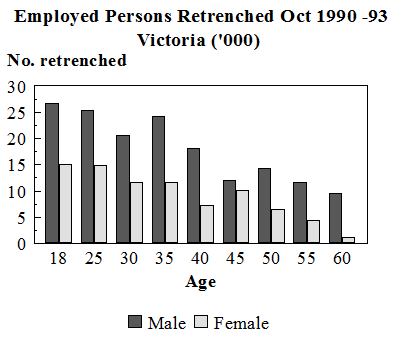Sometimes instead of plotting frequencies we plot relative frequencies which show the percentage of the population within each class interval.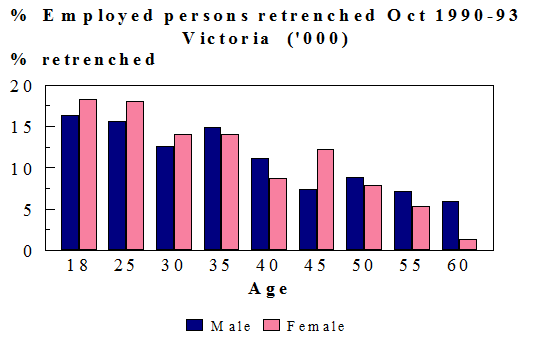Outliers
Summarising the data can be complicated if there are observations which appear to be inconsistent with the remainder of that set of data. It is important to query whether it actually belongs to the data or whether it is a computing, clerical or measurement error. An outlier is an observation that has a major effect on an estimate and which, because of its independently known atypical nature, needs special treatment. Before choosing the treatment for an outlier it is essential to know the reason for its occurrence and whether the outlier provides any information about the population of interest.

Measures of Location (also known as Measures of Central Tendency)
It is often desirable to have summary measures to indicate the location of a frequency distribution on some sort of scale. Often the scale involved in the analysis is a time scale. This helps the researcher build up a picture of the distribution and facilitates some sort of analysis. Summary measures also enable the comparison of frequency distributions before and after a specified event (eg. number of car accidents before and after a change in traffic laws). A change can indicate a shift in the frequency distribution. The most common measures of location are the median, mean and mode.

Mean
The mean is the most commonly used measure of location and is the average of a set of sample or population values. The formulae for calculating population and sample means are:

Population Mean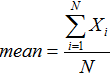where Xi is the observed value of the ith member of the population and N is the total population count.

Sample Mean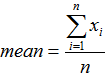where xi is the observed value of the ith member of the sample and n is the total number of units in the sample.
For example, the mean of the set of numbers from a sample: 2, 7, 9, 11, 14 is 8.6.

The mean is used in many statistical tests (e.g. testing differences between groups) and it is possible to calculate standard errors and construct confidence intervals for the mean. In general it is the most stable measure of location but can be badly affected by extreme values.

Median
The median is the middle value when values are sorted into order of size. If there is an even number of values in the set, the median is the average of the middle two values, for example, the median of the set of numbers: 2, 7, 9, 11, 14 is 9; while the median of the set of numbers: 2, 6, 7, 9, 11, 14 is 8. It is a good measure of location for non-symmetrical data as, in such cases, it is more central than the mean and is not affected by extreme values. It is often used in social science research, particularly in areas of housing prices and income. When analysing samples it is difficult to construct confidence intervals for the median due to the complexities in defining the sampling distribution (the distribution of the estimate of median over a number of samples).

Percentiles
A measure of location that is linked to the median is the concept of percentiles. A percentile is a value at or below which a given percentage of the data lies. The 50th percentile is also the median as one half of the population lies below it. Two other important percentiles are the 25th percentile, known as the lower quartile boundary and the 75th percentile, known as the upper quartile boundary. The lower and upper quartile boundaries for the set of numbers: 2, 6, 7, 9, 11, 14 are 6 and 11. Percentiles can only be formed for quantitative variables.

Splitting the population into percentiles enables some comparison of the characteristics of units in each percentile. For example, the average annual income of wage and salary earners in each quartile could be compared, rather than calculating one overall average.

Percentiles are also very useful for comparing changes in characteristics of a population over time. For example, by forming income quartiles for 1986 and 1990 we can determine whether the income share of wage and salary earners in each quartile has changed over time.

Mode
The mode of a frequency distribution is the most frequently occurring value. The mode, however, is not necessarily unique and this can cause problems in measuring the 'centre point' of our values. Having a measure of centre that is not required to take only one value can tell us more about the data than a measure like the mean or the median. In general the mean and median are better measures of location, however the mode is useful when the values are unevenly spread (eg. a two-peak distribution).

Measures of Spread (also known as Measures of Variation)
When summarising datasets it is also important to know the variability (spread) of the values, ie. how spread out the values are around the 'centre'. A measure of location does not provide us with this information so it has to be supplemented with a measure of spread. The common measures of variability are the range, variance and standard error or standard deviation.

Range
The range is the difference between the largest and smallest value. It is a common measure in industrial quality and meteorology because of its ease of computation. However a disadvantage of the range is that it tends to increase as sample size increases and does not provide any information about the distribution of values within the range. The range is also badly affected by extreme values. However, it can be useful for samples from small populations.

The Interquartile Range
The interquartile range, the difference between the upper (75%) and lower (25%) quartile boundaries, covers the 'middle half' of the population. Because it does not take in the ends of the sample distribution, it is not badly affected by outliers. The interquartile range is more difficult to calculate than the range as the data has to be ranked and the quartiles calculated. The interquartile range can be used in editing to create cut-offs when deciding upon which units will be followed up to have their responses verified.

Variance
The variance describes the spread of data around its mean and is the average of the squared differences between the value of the variable (eg. height of each person) and the mean (average height). The more the data is spread the larger the differences will be and therefore the larger the variance will be. It is possible to have two populations with the same mean but different variances. A larger variance indicates that the data is more spread out about the mean.

Standard Deviation
The positive square root of the population variance is called the standard deviation. It is a useful measure of dispersion as it is expressed in the same units as the measures of central tendency. When applied to a sampling distribution it is referred to as the standard error.

Sampling Variance
The variance of a sampling distribution is called sampling variance. The sampling variance of a statistic or estimator is simply the variance of its values obtained from all possible samples.

Standard Error
Standard error often refers to the standard deviation of an estimator (statistic). As the value of an estimator is calculated from a particular sample, different samples will give different values of the estimator. Standard error, the positive square root of the sampling variance, measures the spread of all possible values around the mean (expected value) of an estimator. In practice, only one estimate of the "true value" is available, so the standard error can be derived mathematically. Even if the population variance is unknown, as it happens in practice, the standard error can be estimated by using the variance of the sample units. Standard error is used in the construction of confidence intervals.

Coefficient of variation
It is the standard deviation expressed as a percentage of the sample estimate

Correlation coefficient
The term 'correlation' refers to the existence and extent of a linear relationship between two variables. (That is, where the values of each of two variables are plotted against each other, the relationship can be approximated by a straight line.)

The correlation coefficient :
• measures the strength of the (linear) association between two variables
• ranges from +1 to -1 - (a correlation coefficient of 1 or -1 is a perfect correlation, a correlation coefficient of 0 means there is no correlation)
• does not imply causation (ie. that the values of one of the variables causes the other variable to take a specific value)
• the correlation coefficients can be tested for statistical significance under the Normal assumption

Other Estimates
In addition to estimating means it is often of interest to measure other statistics such as totals, proportions, percentiles or even minimum and maximum values. For instance, the total turnover of retail sales for businesses in Australia or the proportion of unemployed in particular regions may be of interest.

Further Information
The statistical analysis can comprise any summarising or presentation of the data from interpreting confidence intervals about basic summary measures calculated from the survey data, to more complex hypothesis testing using such techniques as contingency table analysis, log-linear modelling, regression analysis and time series analysis. Further information about analysis can be obtained from any of the standard texts in your library. However, it is recommended that consultation with experienced statisticians is worthwhile to determine the most appropriate analysis techniques.

TYPES OF DISTRIBUTIONS
Arrangements of the values of a variable is called the distribution of the variable, for example, the percentages of a group of people in different age groups is called the percentage distribution of the variable 'age'. If the actual numbers or frequencies in different age groups are presented instead of percentages then it is called a frequency distribution. Similarly, the distribution which shows the probability that someone will fall into a particular age group is called its probability distribution. Therefore, a probability distribution shows the chance or probability that the value of a variable will lie in different areas within its range. The curve which shows the probability distribution is called a probability density curve.

Many variables follow what is termed a Normal distribution, but other commonly sought data (eg. income) has a lopsided distribution and is said to have a skewed distribution.

The Normal Distribution
For many populations the distributions of values is a specific bell-shaped curve, called the Normal curve. Such curves are characterised by the peak being the mean, median and mode of the distribution. The Normal distribution is the most useful distribution in statistics as most data sets can be approximated normally and nearly all sampling distributions converge to a Normal distribution. Consequently testing of hypotheses and the constructing of confidence intervals is relatively straightforward. Many phenomena in everyday life can be described by the Normal curve, for example, the height of males and females.

In a Normal distribution, 95% of all observations lie within 2 standard deviations either side of the population mean. Similarly, 99% of all observations lie within 3 standard deviations either side of the population mean. For the height example, a small proportion of a population are usually very short or very tall, with the majority falling in some middle range.

Normal Distribution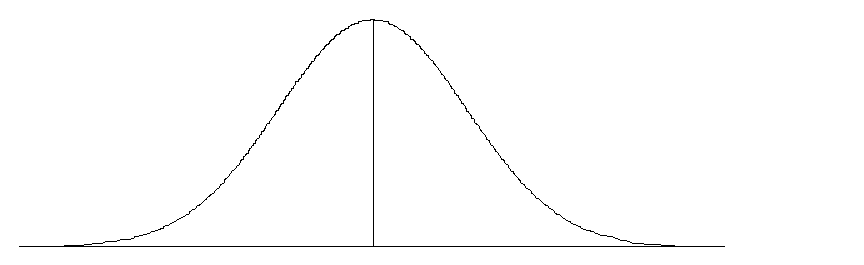Skewed Distributions
Some populations are specified by curves that are non-symmetrical (skewed) in shape. Such curves are characterised by the peak being located to one side of the centre of the data, which causes the tails either side of the peak to be of unequal length and angle. Medians are good measures of 'location' for skewed distributions. Variables such as house prices and income are usually positively skewed.

Positively Skewed Distribution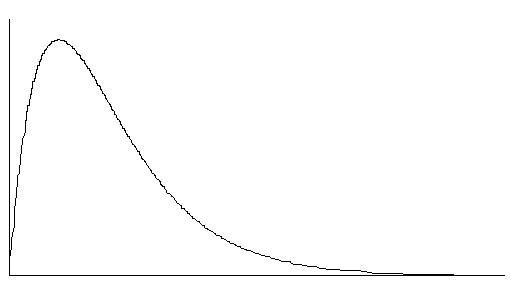ESTIMATION
In a sample survey, we are always required to make estimates of certain population parameters. This is done in order to make inferences about the population as a whole. Good estimators are generally unbiased. In other words, theory indicates that across all possible samples the average sample value is equal to the population value, regardless of the sample size. A good estimator will also have a low variance and thus be very close to the population parameter we wish to estimate no matter which units are included in the sample that we take.

In analysing survey results, we usually require estimates of population total and mean. The two most commonly used methods of estimation are number-raised estimation and ratio estimation.

Weighting
Note that in order to avoid the researcher drawing spurious conclusions, great care must be taken to weight and aggregate the data correctly. Weighting is the process whereby each unit in the sample has its response inflated to represent the response from all similar units in the population. The weight of a unit reflects the proportion of the population that the sampled unit represents. The weight allocated to each sample observation depends on the process used to select the sample. The most simple form of weighting is where a simple random sample (SRS) of size n is selected from a known population of size N.

Suppose we want to estimate total employment for 100 cafe and restaurant businesses located in the City of Melbourne (N=100). Due to resource constraints we can only approach 10 businesses (n=10). Each business has a one in ten chance of selection and each business selected represents itself and 9 other businesses. The weight allocated to each selected business is therefore 10.

Number-Raised Estimation
If we observe a sequence of n observations y1 , ... ,yn from a population of size N, then the number-raised estimator for the population total is the sample total multiplied by the ratio of population size to sample size (N/n).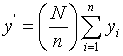Our number-raised estimate is unbiased as the average of all possible samples is the true population total.
The basis of this method is that the average (mean) of a sample is the best estimate of the mean of a population. So if we want to find the average population of Melbourne suburbs we select a representative sample of suburbs and take the average of this sample as our estimate of the average population in Melbourne suburbs. Then to obtain the estimate of the total population in Melbourne, the average population in suburbs is multiplied by the total number of suburbs.

Remember that each business has a one in ten chance of selection. If the total turnover from the sample of 10 cafe and restaurant businesses is \$5 million then the number-raised estimate of total income from the population of cafe and restaurant businesses in the City of Melbourne is \$5 million * 10 = \$50 million.

This form of estimation is easy to use and does not require any benchmark information. It is relatively simple to calculate and its variance formula is known.

Number-raised estimation has problems in that it produces a large sampling error compared to ratio estimation and is badly affected by unrepresentative samples.

Ratio Estimation

Instead of calculating population values from sample values by inflating them by the ratio of the number of population units to the number of sample units, ratio estimation uses a ratio of population to sample totals based on some other variable. For example, it may be useful in a survey of job vacancies to use a ratio of total employment in the stratum to total employment in the selected firms, rather than simply the ratio of total number of firms to selected number of firms. This other variable is known as the benchmark or auxiliary variable. For it to be effective, this variable should be highly correlated with our variable of interest and needs to be known for all units in the population. If we define yi to be our variable of interest and xi as our benchmark variable, then our ratio estimate is: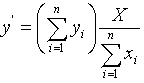(where X is the population total for the auxiliary variable).

The average of all possible sample estimates will not be exactly equal to the true value. Thus our ratio estimate is biased.

Total employment was known to be a useful auxiliary variable in estimating total turnover of the cafe and restaurant businesses in the City of Melbourne and is known for every business in the population. The total employment for the population was found to be 1,500 people and the total employment for the sample was found to be 100 people. The calculation of the estimate of total turnover from the population uses the ratio of population to sample totals based on total employment (1,500 /100 = 15) as its weight. The ratio estimate of total turnover for cafe and restaurant businesses in the City of Melbourne is therefore;

\$5 million * 15 = \$75 million.

The value of ratio estimation is that it decreases the standard errors of the estimates when the benchmark variable is highly correlated with the variable of interest. The ratio estimate also remains relatively unaffected by unrepresentative samples.